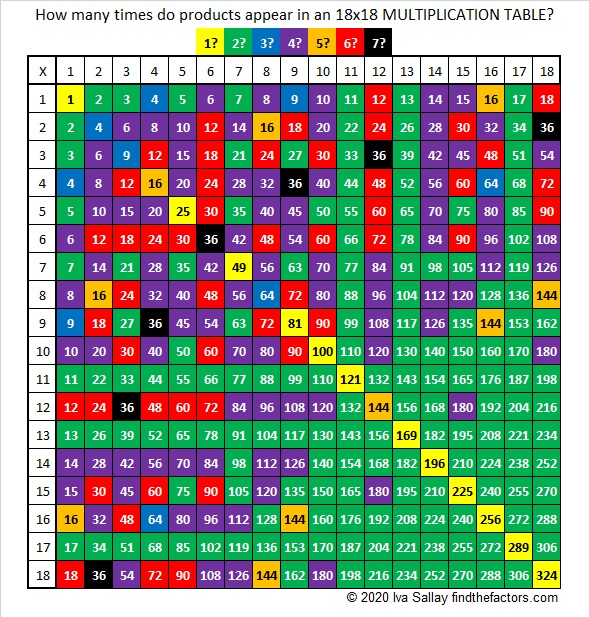# 1557 Happy Birthday to My Brother, Andy!

Contents

### Today’s puzzle:

Today is my brother Andy’s birthday. He enjoys solving puzzles so I made this 18 × 18 puzzle hoping that he will find it challenging.This 18 × 18 multiplication table will be invaluable as you work to solve it. When you look for a clue in the table, its color will let you know how many times it appears in the table.Print the puzzles or type the solution in this excel file: 10 Factors 1546-1557.

If you need a hint to solve the puzzle: One of the first things you will want to do is identify the clues that are multiples of 5, 10, or 15. Then use logic to determine which clues will use the two 5’s, the two 10’s, and the two 15’s.

### Factors of 1557:

• 1557 is a composite number.
• Prime factorization: 1557 = 3 × 3 × 173, which can be written 1557 = 3² × 173.
• 1557 has at least one exponent greater than 1 in its prime factorization so √1557 can be simplified. Taking the factor pair from the factor pair table below with the largest square number factor, we get √1557 = (√9)(√173) = 3√173.
• The exponents in the prime factorization are 2 and 1. Adding one to each exponent and multiplying we get (2 + 1)(1 + 1) = 3 × 2 = 6. Therefore 1557 has exactly 6 factors.
• The factors of 1557 are outlined with their factor pair partners in the graphic below.### More about the number 1557:

From OEIS.org we learn that 1557 has a rather fun square:
1557² = 2424249.

1557 is the sum of two squares:
39² + 6² = 1557.

1557 is the hypotenuse of a Pythagorean triple:
468-1485-1557, which is 9 times (52-165-173).
It can also be calculated from 2(39)(6), 39² – 6², 39² + 6².

1557 is also the difference of two squares three different ways:
779² – 778² = 1557,
261² – 258² = 1557, and
91² – 82² = 1557.

This site uses Akismet to reduce spam. Learn how your comment data is processed.